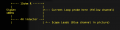# oscilloscope measuring current/voltage phase shift

#### chicken-little

Joined Jun 26, 2021
2
Hello, I am trying to demonstrate current/voltage phase shift with an oscilloscope. I have a current probe and a regular probe for voltage. A simple circuit from a signal generator to a resistor and inductor in series. Measuring across resistor I see both I/V are in phase. What am I doing wrong? How can I demonstrate phase shift? Thanks much!

#### AlbertHall

Joined Jun 4, 2014
12,199
Measuring across resistor I see both I/V are in phase.
The voltage and current through a resistor will always be in phase. Measure across the inductor instead, or at the output of the signal generator.

#### chicken-little

Joined Jun 26, 2021
2
Well let me add more details.Signal Gen reads 10V(Amplitude) ie. 10*0.7071 = 7V rms

Inductor coil measured with L meter at 100Hz freq. show 4 Henries

Current probe set to 10mA per 1mV. Scope shows 14mV peak-peak, ie 5mV RMS => 50mA

Reactance Xl = 2*pi*100*4 = 2400ohm
Z = sqrt(sqr(15) + sqr(2400))
= 2400.04ohm

140mA(p-p) is 70mA peak is 70ma * 0.7071 = 49.5mA

V = I*Z
= 0.05*2400
= 120V !!?? # Expecting 7V rms (10V amplitude * 0.7071 = 7V RMS)

The phase angle (phi) between the voltage and the current.

tan(phi) = Xl/R = 2400/15 = 160
phi = arctan(160) = 89.6deg

Can someone explain the strange curves on the scope??
View attachment phase-shift.jpg

#### AlbertHall

Joined Jun 4, 2014
12,199

#### du00000001

Joined Nov 10, 2020
114
chicken-little said:
Well let me add more details.
...
Well, would be nice if you could add some more details - for starters a schematic clearly indicating what's connected where and how.
Currently your scope picture is kind of mystery for me: I can see that following every zero-crossing the current is zero until some device (Schottky diode?) seems to start to conduct. First I thought this might be the generator, but now I don't. Would be nice if you could check the generator's output - loaded and unloaded. Just a simple voltage curve...